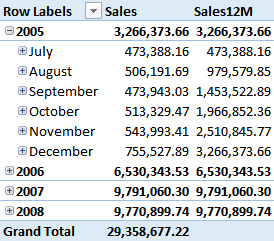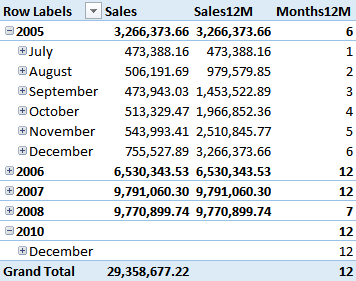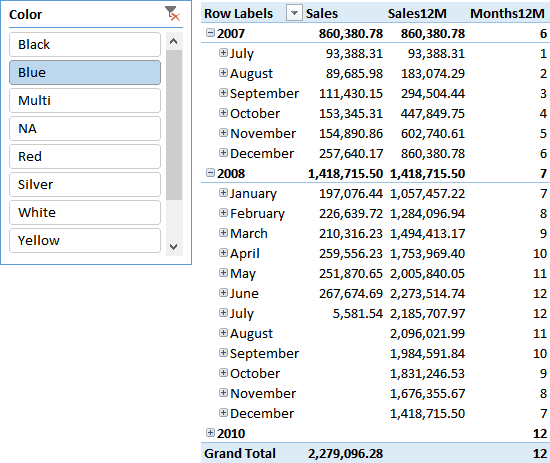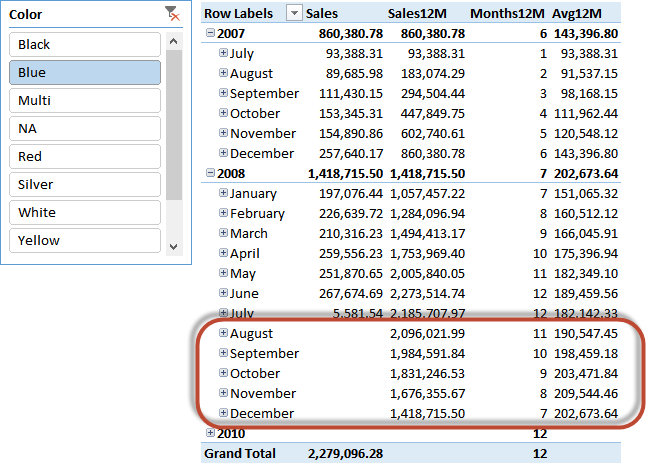We start with the usual AdventureWorks data model, with the Products, Sales and Calendar tables. Calendar has been marked as a calendar table as it is necessary to work with any time intelligence function, and we built a simple year-month-date hierarchy. With this set up, it is very easy to create a first PivotTable showing sales over time:When doing trend analyses, if sales are subject to seasonality or more generally if you want to remove the effect of peaks and drops in sales, a common technique is that of computing the value over a given period, usually 12 months, and average it. The rolling average over 12 months provides a smooth indicator of the trend and it is very useful in charts.

Given a date, we can compute the rolling 12-months average with the following formula. It does involve a few challenges that we will solve later:

```Sales12M := CALCULATE (
[Sales],
DATESBETWEEN (
Calendar[FullDate],
SAMEPERIODLASTYEAR ( NEXTDAY ( LASTDATE ( Calendar[FullDate] ) ) ),
LASTDATE ( Calendar[FullDate] )
)
)
```

The formula’s behavior is simple: it computes the value of [Sales] after creating a filter on the calendar that shows exactly one full year worth of data. The core of the formula is the DATESBETWEEN formula, which returns an inclusive set of dates between the two boundaries. The lower boundary is:

```SAMEPERIODLASTYEAR ( NEXTDAY ( LASTDATE ( Calendar[FullDate] ) ) )
```

Reading it from the innermost step out: if we are showing data for a month, say July 2007, we take the last visible date using LASTDATE, which returns the last day in July 2007. We use then NEXTDAY to return August 1st, 2007 and we finally use SAMEPERIODLASTYEAR to shift it back one year, yielding August 1st, 2006. The upper boundary is simply LASTDATE, i.e. end of July 2007.

If we use this formula in a PivotTable, the result looks fine, but we have a problem for the last date:As you can see in the PivotTable above, the value is computed correctly until 2008. Then, there is no value in 2009 — which is correct, as we don’t have sales in 2009 — but there is a surprising value in December 2010, where our formula shows the grand total instead of the blank value we would expect.

In fact, in December, LASTDATE returns the last day of the year and NEXTDAY should return January 1st, 2011. But NEXTDAY is a time intelligence function and it is expected to return sets of existing dates. This is not the easiest concept to grasp and it is worth explaining further.

Time intelligence functions do not perform math on dates. If you want to take the day after a given date, you can simply add 1 to any date column, and the result will be the next day. Instead, time intelligence functions shift sets of dates back and forth over time. Thus, NEXTDAY takes its input (in our case a single-row table with December 31st, 2010) and shifts it one day later. The problem is that though the result should be January 1st, 2011, the result is BLANK because the Calendar table does not contain that date.

Thus, our expression computes [Sales] with a blank lower boundary, which means the beginning of time, yielding the grand total of sales. To correct the formula it is enough to change the evaluation order of the lower boundary:

```Sales12M := CALCULATE (
[Sales],
DATESBETWEEN (
Calendar[FullDate],
NEXTDAY ( SAMEPERIODLASTYEAR ( LASTDATE ( Calendar[FullDate] ) ) ),
LASTDATE ( Calendar[FullDate] )
)
)
```

As you can see, now NEXTDAY is called after the one-year back shift. We take December 31st, 2010, move it to December 31st, 2009 and take the next day, which is January 1st, 2010: a date that actually exists in the calendar table.

The result is now as expected:At this point, we only need to divide that number by 12 to obtain the rolling average. But, as you can easily imagine, we cannot always divide it by 12. In fact, at the beginning of the period there are not 12 months to aggregate, but fewer. We need to compute the number of months for which there are sales. This can be accomplished using cross filtering of the calendar table with the sales table after applying the new 12-months context. This is how We define a new measure computing the number of existing months in the 12-months period:

```Months12M := CALCULATE (
CALCULATE ( COUNTROWS ( VALUES ( Calendar[MonthName] ) ), Sales ),
DATESBETWEEN (
Calendar[FullDate],
NEXTDAY ( SAMEPERIODLASTYEAR (LASTDATE ( Calendar[FullDate] ) ) ),
LASTDATE ( Calendar[FullDate] )
)
)
```

You can see in the next figure that the Months12M measure computes correctly:It is worth noting that the formula does not work if you choose a period longer than 12 months, because Calendar[MonthName] only has 12 values. If you need longer periods, you will need to use an YYYYMM column to be able to count to more than 12.

The interesting part of this formula that uses cross filtering is the fact that it computes the number of available months even when you filter using other attributes. If, for example, you select the Blue color using a slicer, then sales start in July 2007 — not in 2005, as for many other colors. Using the cross filter on Sales, the formula correctly computes that in July 2007 there is a single month of available sales for Blue:At this point, the rolling average is just a DIVIDE away:

```Avg12M := DIVIDE ( [Sales12M], [Months12M] )
```

When we use this measure in a Pivot Table, we still have a small issue: the value is computed also for months without sales (i.e. future months):This could be solved using an IF statement to prevent the formula from showing values when there are no sales. However, and I have nothing against IF — for the performance-addicted among you remember that IF might be a performance killer because it could force the DAX formula engine to kick in. In this specific case, the difference is negligible. However, as a general rule, the best way to remove the value when there are no sales is to rely on pure storage engine formulas like this one:

```Avg12M := DIVIDE ( [Sales], [Sales] ) * DIVIDE ( [Sales12M], [Months12M] )
```

Comparing a Rolling 12-Months Average of Sales chart using the Avg12M with a Sales chart you can easily appreciate how the rolling average outlines trends in a much more smoothly: